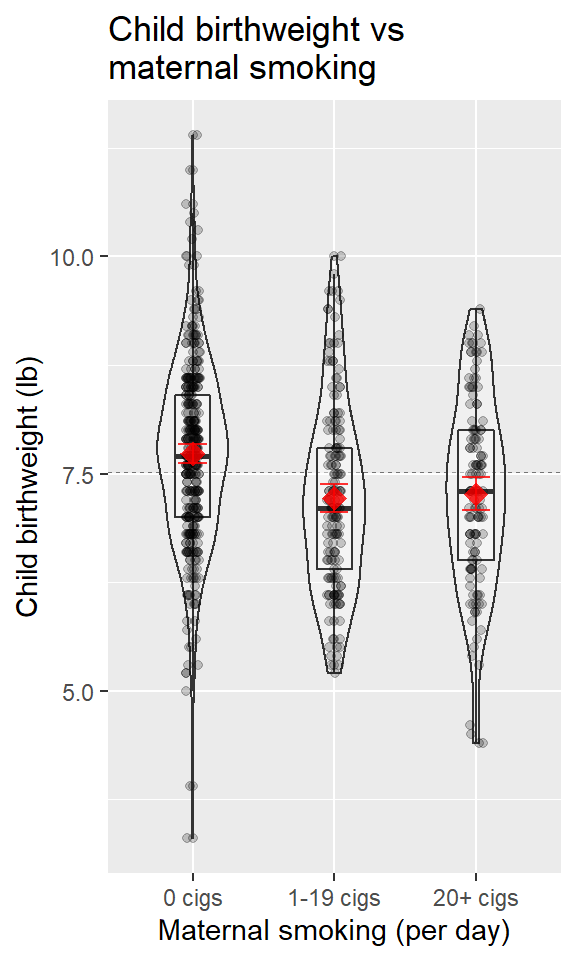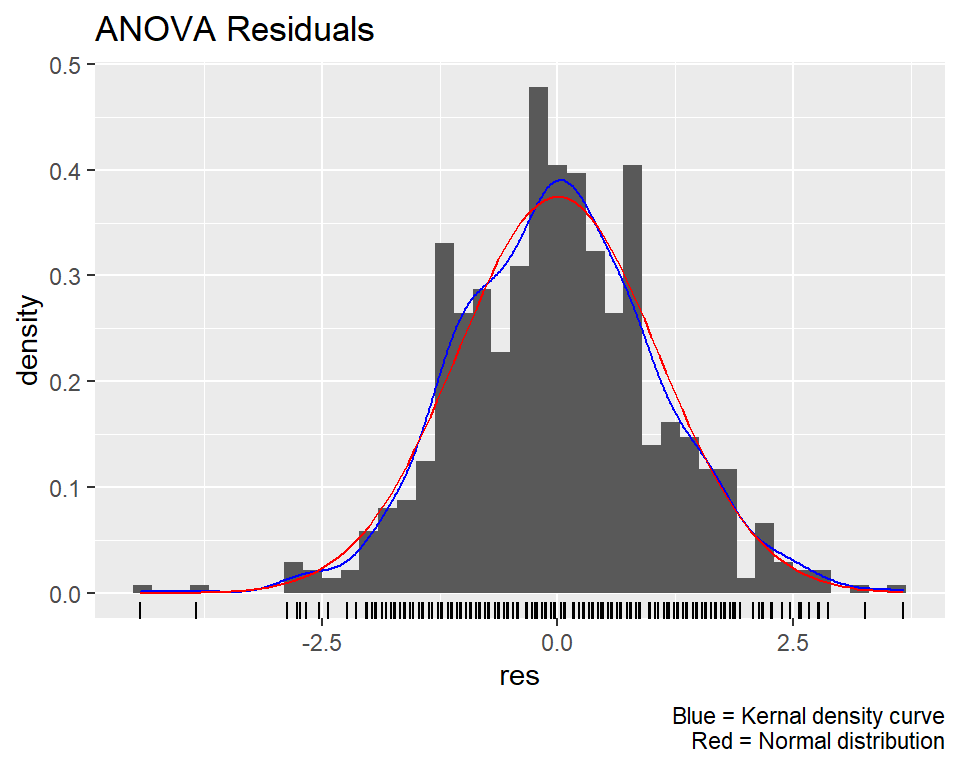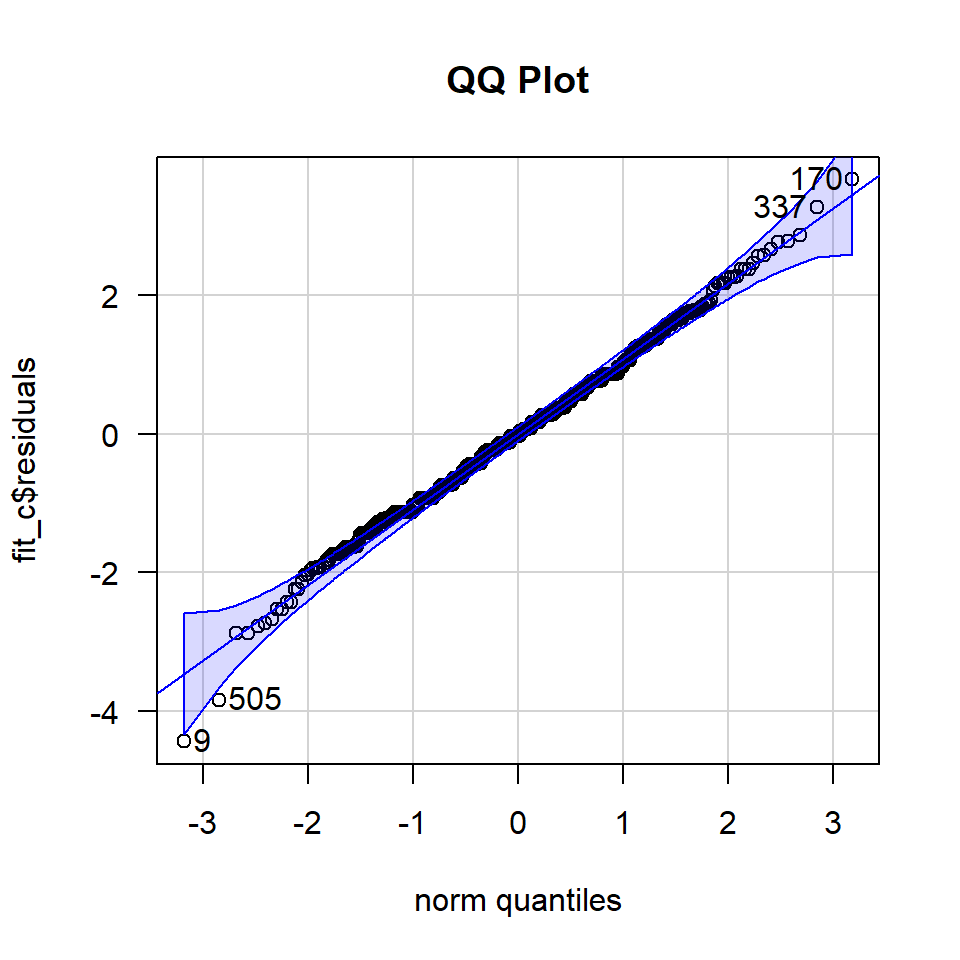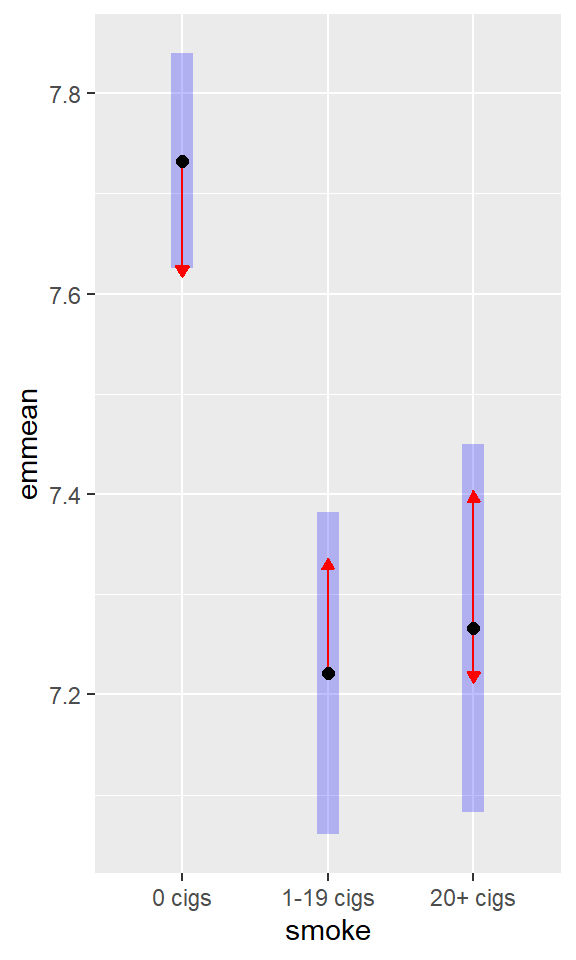# ADA1: Class 21, ANOVA, Pairwise comparisons

Advanced Data Analysis 1, Stat 427/527, Fall 2022, Prof. Erik Erhardt, UNM

Author

Published

August 13, 2022

Include your answers in this document in the sections below the rubric where I have point values indicated (1 p).

# Rubric

Answer the questions with the data example.

# Example: Child Health and Development Study (CHDS)

We consider data from the birth records of 680 live-born white male infants. The infants were born to mothers who reported for pre-natal care to three clinics of the Kaiser hospitals in northern California. As an initial analysis, we will examine whether maternal smoking has an effect on the birth weights of these children. To answer this question, we define 3 groups based on mother’s smoking history:

1. mother does not currently smoke or never smoked,
2. mother smoked less than one pack of cigarettes a day during pregnancy, and
3. mother smoked at least one pack of cigarettes a day during pregnancy.

Let $$\mu_i$$ = pop mean birth weight (lb) for children in group $$i$$, $$(i=1,2,3)$$. We wish to test $$H_0: \mu_1 = \mu_2 = \mu_3$$ against $$H_A: \textrm{not } H_0$$.

We read in the data, create a smoke factor variable, and plot the data by smoking group.

library(tidyverse)
── Attaching packages ─────────────────────────────────────── tidyverse 1.3.2 ──
✔ ggplot2 3.3.6     ✔ purrr   0.3.4
✔ tibble  3.1.8     ✔ dplyr   1.0.9
✔ tidyr   1.2.0     ✔ stringr 1.4.0
✔ readr   2.1.2     ✔ forcats 0.5.1
── Conflicts ────────────────────────────────────────── tidyverse_conflicts() ──
✖ dplyr::lag()    masks stats::lag()
#### Example from the Child Health and Development Study (CHDS)
dat_chds <-
read_csv("ADA1_notes_05-CHDS.csv")
Rows: 680 Columns: 13
── Column specification ────────────────────────────────────────────────────────
Delimiter: ","
chr (5): id, m_smok, m_ppwt, p_educ, p_smok
dbl (8): c_head, c_len, c_bwt, gest, m_age, m_ht, p_age, p_ht

ℹ Use spec() to retrieve the full column specification for this data.
ℹ Specify the column types or set show_col_types = FALSE to quiet this message.
dat_chds <-
dat_chds %>%
mutate(
# create a factor variable based on number of cigarettes smoked
smoke = case_when(
# no cigs
m_smok == 0                           ~ "0 cigs"
# less than 1 pack (20 cigs = 1 pack)
, (m_smok > 0) & (dat_chds$m_smok < 20) ~ "1-19 cigs" # at least 1 pack (20 cigs = 1 pack) , m_smok >= 20 ~ "20+ cigs" ) , smoke = factor(smoke) ) %>% select( c_bwt , smoke ) summary(dat_chds)  c_bwt smoke Min. : 3.300 0 cigs :381 1st Qu.: 6.800 1-19 cigs:169 Median : 7.600 20+ cigs :130 Mean : 7.516 3rd Qu.: 8.200 Max. :11.400  Plot the data in a way that compares the means. Error bars are 95% confidence intervals of the mean. # Plot the data using ggplot library(ggplot2) p <- ggplot(dat_chds, aes(x = smoke, y = c_bwt)) # plot a reference line for the global mean (assuming no groups) p <- p + geom_hline(yintercept = mean(dat_chds$c_bwt),
colour = "black", linetype = "dashed", size = 0.3, alpha = 0.5)
# boxplot, size=.75 to stand out behind CI
p <- p + geom_violin(width = 0.5, alpha = 0.25)
p <- p + geom_boxplot(width = 0.25, alpha = 0.25)
# points for observed data
p <- p + geom_point(position = position_jitter(w = 0.05, h = 0), alpha = 0.2)
# diamond at mean for each group
p <- p + stat_summary(fun = mean, geom = "point", shape = 18, size = 4,
colour = "red", alpha = 0.8)
# confidence limits based on normal distribution
p <- p + stat_summary(fun.data = "mean_cl_normal", geom = "errorbar",
width = .2, colour = "red", alpha = 0.8)
p <- p + labs(x     = "Maternal smoking (per day)"
, y     = "Child birthweight (lb)"
, title = "Child birthweight vs\nmaternal smoking"
)
print(p)Hypothesis test

1. Set up the null and alternative hypotheses in words and notation.

• In words: The population mean birthweight is different between smoking groups.’’
• In notation: $$H_0: \mu_1=\mu_2=\mu_3$$ versus $$H_A: \textrm{not } H_0$$ (at least one pair of means differ).
2. Let the significance level of the test be $$\alpha=0.05$$.

3. Compute the test statistic.

fit_c <-
aov(
c_bwt ~ smoke
, data = dat_chds
)
summary(fit_c)
             Df Sum Sq Mean Sq F value   Pr(>F)
smoke         2   40.7  20.351    17.9 2.65e-08 ***
Residuals   677  769.5   1.137
---
Signif. codes:  0 '***' 0.001 '**' 0.01 '*' 0.05 '.' 0.1 ' ' 1

The $$F$$-statistic for the ANOVA is $$F = 17.9$$.

1. Compute the $$p$$-value from the test statistic.

The p-value for testing the null hypothesis is $$p = 2.65\times 10^{-8}$$.

1. (2 p) State the conclusion in terms of the problem.

1. Check assumptions of the test.
1. Residuals are normal
2. Populations have equal variances.
• Check whether residuals are normal.

• Plot the residuals and assess whether they appear normal.

# Plot the data using ggplot
df_res <- data.frame(res = fit_c$residuals) library(ggplot2) p <- ggplot(df_res, aes(x = res)) p <- p + geom_histogram(aes(y = ..density..), binwidth = 0.2) p <- p + geom_density(colour = "blue") p <- p + geom_rug() p <- p + stat_function(fun = dnorm, colour = "red", args = list(mean = mean(df_res$res), sd = sd(df_res$res))) p <- p + labs(title = "ANOVA Residuals" , caption = "Blue = Kernal density curve\nRed = Normal distribution") print(p)(1 p) Describe the plot of residuals as it relates to model assumptions. [ANSWER HERE] • Plot the residuals versus the normal quantiles. If the residuals are normal, then the will fall on the center line and very few will be outside the error bands. # QQ plot par(mfrow=c(1,1)) #library(car) car::qqPlot( fit_c$residuals
, las = 1
, id = list(n = 4, cex = 1)
, lwd = 1
, main="QQ Plot"
)9 505 170 337

(1 p) Describe the plot of residuals as it relates to model assumptions.

• A formal test of normality on the residuals tests the hypothesis $$H_0:$$ The distribution is Normal vs $$H_1:$$ The distribution is not Normal. We can test the distribution of the residuals.

Three tests for normality are reported below. I tend to like the Anderson-Darling test. Different tests have different properties, and tests that are sensitive to differences from normality in the tails of the distribution are typically more important for us (since deviations in the tails are more influential than deviations in the center).

shapiro.test(fit_c$residuals)  Shapiro-Wilk normality test data: fit_c$residuals
W = 0.99553, p-value = 0.04758
#library(nortest)
nortest::ad.test(fit_c$residuals)  Anderson-Darling normality test data: fit_c$residuals
A = 0.62184, p-value = 0.1051
nortest::cvm.test(fit_c$residuals)  Cramer-von Mises normality test data: fit_c$residuals
W = 0.091963, p-value = 0.1449

(1 p) Interpret the conclusion of the Anderson-Darling test.

• Check whether populations have equal variances.

• Look at the numerical summaries below.

# calculate summaries
dat_chds_summary <-
dat_chds %>%
group_by(smoke) %>%
summarize(
m = mean(c_bwt)
, s = sd(c_bwt)
, n = n()
, .groups = "drop_last"
) %>%
ungroup()

dat_chds_summary
# A tibble: 3 × 4
smoke         m     s     n
<fct>     <dbl> <dbl> <int>
1 0 cigs     7.73  1.05   381
2 1-19 cigs  7.22  1.08   169
3 20+ cigs   7.27  1.09   130

(1 p) Interpret the standard deviations above. You may also discuss the plots of the data.

• Formal tests for equal variances. We can test whether the variances are equal between our three groups. This is similar to the ANOVA hypothesis, but instead of testing means we’re tesing variances. $$H_0: \sigma^2_1=\sigma^2_2=\sigma^2_3$$ versus $$H_A: \textrm{not } H_0$$ (at least one pair of variances differ).
## Test equal variance
# assumes populations are normal
bartlett.test(c_bwt ~ smoke, data = dat_chds)

Bartlett test of homogeneity of variances

data:  c_bwt by smoke
Bartlett's K-squared = 0.3055, df = 2, p-value = 0.8583
# does not assume normality, requires car package
#library(car)
car::leveneTest(c_bwt ~ smoke, data = dat_chds)
Levene's Test for Homogeneity of Variance (center = median)
Df F value Pr(>F)
group   2  0.7591 0.4685
677               
# nonparametric test
fligner.test(c_bwt ~ smoke, data = dat_chds)

Fligner-Killeen test of homogeneity of variances

data:  c_bwt by smoke
Fligner-Killeen:med chi-squared = 2.0927, df = 2, p-value = 0.3512

(1 p) Interpret the result of the appropriate test. If normality was reasonable then use Bartlett, otherwise use Levene.

1. If the ANOVA null hypothesis was rejected, then perform follow-up Post Hoc pairwise comparison tests to determine which pairs of means are different.

There are several multiple comparison methods described in the notes. Let’s use Tukey’s Honest Significant Difference (HSD) here to test which pairs of populations differ.

EMM plot interpretation

This EMM plot (Estimated Marginal Means, aka Least-Squares Means) is only available when conditioning on one variable. The blue bars are confidence intervals for the EMMs; don’t ever use confidence intervals for EMMs to perform comparisons – they can be very misleading. The red arrows are for the comparisons among means; the degree to which the “comparison arrows” overlap reflects as much as possible the significance of the comparison of the two estimates. If an arrow from one mean overlaps an arrow from another group, the difference is not significant, based on the adjust setting (which defaults to “tukey”).

## CHDS
# Tukey 95% Individual p-values
#TukeyHSD(fit_c)

## Contrasts

library(emmeans)
emm_cont <-
emmeans::emmeans(
fit_c
, specs = "smoke"
)

# means and CIs
emm_cont %>% print()
 smoke     emmean     SE  df lower.CL upper.CL
0 cigs      7.73 0.0546 677     7.63     7.84
1-19 cigs   7.22 0.0820 677     7.06     7.38
20+ cigs    7.27 0.0935 677     7.08     7.45

Confidence level used: 0.95 
# pairwise differences
emm_cont %>% pairs(adjust = adjust_method) %>% print()
 contrast                 estimate     SE  df t.ratio p.value
0 cigs - (1-19 cigs)       0.5115 0.0985 677   5.191  <.0001
0 cigs - (20+ cigs)        0.4667 0.1083 677   4.309  0.0001
(1-19 cigs) - (20+ cigs)  -0.0449 0.1244 677  -0.361  0.9308

P value adjustment: tukey method for comparing a family of 3 estimates 
# plot of means, CIs, and comparison arrows
plot(
emm_cont
, comparisons = TRUE
, horizontal = FALSE
, ylab = "smoke"
)(2 p) Interpret the comparisons (which pairs differ).

• 0 cigs - (1-19 cigs): means are (not) significant different
• 0 cigs - (20+ cigs): means are (not) significant different
• (1-19 cigs) - (20+ cigs): means are (not) significant different

(1 p) Summarize results by ordering the means and grouping pairs that do not differ (see notes for examples).

Replace this example with your results.
-----------------